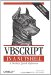# Section C.3. Comparison Operators

### C 3 Comparison Operators

There are three main comparison operators: < (less-than), > (greater-than), and = (equal to). They can be used individually, or any two operators can be combined with each other. Their general syntax is:

`result = expression1 operator expression2 `

The resulting expression is True (-1), False (0), or Null. A Null results only if either expression1 or expression2 itself is Null.

What follows is a list of all the comparison operators supported by VBScript, as well as an explanation of the condition required for the comparison to result in True:

>

expression1 is greater than and not equal to expression2

<

expression1 less than and not equal to expression2

<> or ><

expression1 not equal to expression2 (less than or greater than)

>= or =>

expression1 greater than or equal to expression2

<= or =<

expression1 less than or equal to expression2

=

expression1 equal to expression2

Comparison operators can be used with both numeric and string variables. Literal numbers and strings are called hard. Variables and other expressions are called soft. When comparing two expressions where one is a string and one is a numeric, the rules are:

1. If both are hard, the string is converted to a number before the comparison is executed.
2. If one is hard and one is soft, then the soft one is converted to the type of the hard one before the comparison.
3. If both are soft, then the number will be considered "smaller" than the string.

C.3.1 The Is Operator

The Is operator determines whether two object reference variables refer to the same object. Thus, it tests for the "equality" of two object references. Its syntax is:

`result = object1 Is object2`

If both object1 and object2 refer to the same object, the result is True; otherwise, the result is False. You also use the Is operator to determine whether an object variable refers to a valid object. This is done by comparing the object variable to the special Nothing value:

`If oVar Is Nothing Then`

The result is True if the object variable does not hold a reference to an object.VBScript in a Nutshell, 2nd Edition
ISBN: 0596004885
EAN: 2147483647
Year: 2003
Pages: 335

Similar book on Amazon